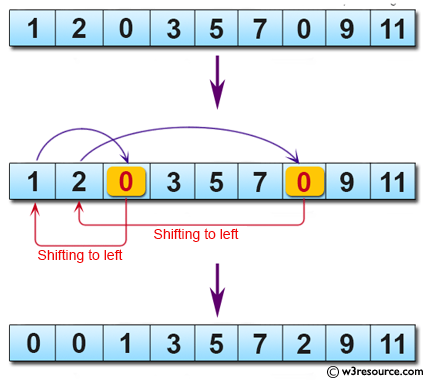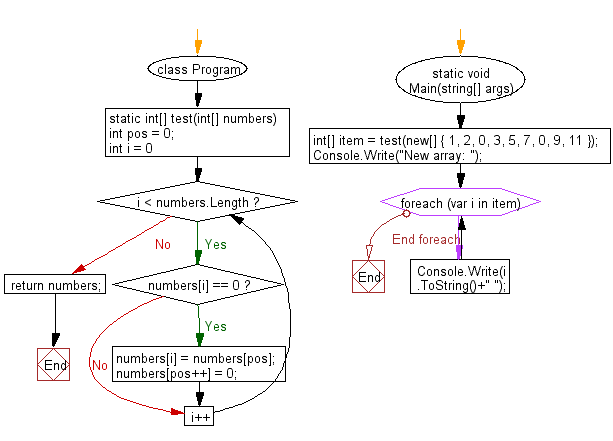﻿ C# - Shift all zeros to the left from an array of integers# C# Sharp Basic Algorithm Exercises: Create a new array from a given array of integers shifting all zeros to left direction by swapping with number

## C# Sharp Basic Algorithm: Exercise-130 with Solution

Write a C# Sharp program to create a new array from a given array of integers shifting all zeros to left direction by swapping with number.

Pictorial Presentation:Sample Solution:

C# Sharp Code:

``````using System;
namespace exercises
{
class Program
{
static void Main(string[] args)
{
int[] item = test(new[] { 1, 2, 0, 3, 5, 7, 0, 9, 11 });
Console.Write("New array: ");
foreach(var i in item)
{
Console.Write(i.ToString()+" ");
}
}
static int[] test(int[] numbers)
{
int pos = 0;
for (int i = 0; i < numbers.Length; i++)
{
if (numbers[i] == 0)
{
numbers[i] = numbers[pos];
numbers[pos++] = 0;
}
}
return numbers;
}
}
}
```
```

Sample Output:

`New array: 0 0 1 3 5 7 2 9 11`

Flowchart:C# Sharp Code Editor:

Improve this sample solution and post your code through Disqus

What is the difficulty level of this exercise?

Test your Programming skills with w3resource's quiz.

﻿